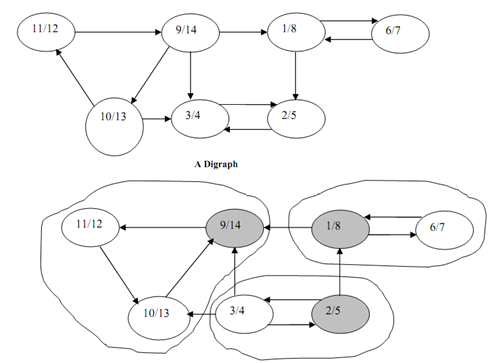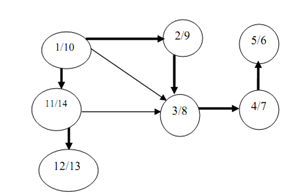## Find strongly connected components - dfs, Data Structure & Algorithms

Assignment Help:

A striking application of DFS is determine a strongly connected component of a graph.

Definition: For graph G = (V, E) , where V refer to the set of vertices and E refer to the set of edges, we described a strongly connected components as follows:

U refers to a sub set of V such that u, v linked to U such that, there is a path from u to v and v to u. That is, all of the pairs of vertices are reachable from each other.

In this section we shall use another concept called transpose of any graph. Given a directed graph G a transpose of G is described as GT. GT is described as a graph along with the same number of vertices & edges with only the direction of the edges being reversed. GT is attained by transposing the adjacency matrix of the directed graph G.

The algorithm for determining these strongly connected components uses the transpose of G, GT.

G = ( V, E ), GT = ( V, ET ), where ET = {  ( u, v ): ( v, u ) belongs to E }Figure: Transpose and strongly connected components of digraph of above Figure

Figure illustrates a directed graph along with sequence in DFS (first number of the vertex illustrates the discovery time and second number illustrates the finishing time of the vertex during DFS. Figure illustrates the transpose of the graph in Figure whose edges are reversed. The strongly connected components are illustrated in zig-zag circle in Figure.To determine strongly connected component we begun with a vertex with the highest finishing time and begun DFS in the graph GT and then in decreasing order of finishing time. DFS along vertex with finishing time 14 as root determine a strongly connected component. Alike, vertices along finishing times 8 and then 5, while chosen as source vertices also lead to strongly connected components.

#### Pipeling, Asktypes of pipelining question #Minimum 100 words accepted#

Asktypes of pipelining question #Minimum 100 words accepted#

#### Kruskal algorithm for minimum spanning, Implementations of Kruskal's algori...

Implementations of Kruskal's algorithm for Minimum Spanning Tree. You are implementing Kruskal's algorithm here. Please implement the array-based Union-Find data structure.

#### Stack, using a program flowchart design a program to illustrate pop and pus...

using a program flowchart design a program to illustrate pop and push operation

#### Compound interest, Write the algorithm for compound interest

Write the algorithm for compound interest

#### Total weight of minimum spanning tree, a) Run your program for α = 0.05, 0...

a) Run your program for α = 0.05, 0.5, and 0.95. You can use n = 30, and W = 10. What is impact of increasing value of α on connectivity of G'? To answer this question, for each v

#### What is a container taxonomy, What is A Container Taxonomy It's useful ...

What is A Container Taxonomy It's useful to place containers in a taxonomy to help understand their relationships to one another and as a basis for implementation using a class

#### Binary search tree in ascending order, In order to get the contents of a Bi...

In order to get the contents of a Binary search tree in ascending order, one has to traverse it in In-order

#### Implement an algorithm to simulate car re-organizing, Design  and implement...

Design  and implement  an algorithm  to simulate car  re-organizing of the train at the railway switching junction. You can only use stacks as the data structure to represent the t

#### Explain time complexity, Time Complexity:- The time complexity of an algori...

Time Complexity:- The time complexity of an algorithm is the amount of time it requires to run to completion. Some of the reasons for studying time complexity are:- We may be in

#### Tree traversal, Q. What do you understand by the tree traversal? Write down...

Q. What do you understand by the tree traversal? Write down the procedure for traversing a binary tree in preorder and execute it on the following tree.    Ans: Th